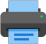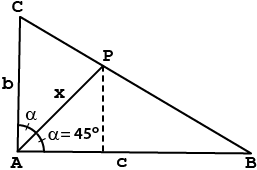Lista de exercícios do ensino médio para impressãoSelect all.
print selected exercises
Knowing that in the right triangle ABC shown in the picture b = 1 and c = 2, find x.
a)
$\,\sqrt{2}\,$
b)
$\,\dfrac{3}{2}\,$
c)
$\,\dfrac{3\sqrt{2}}{2}\,$
d)
$\,\dfrac{2}{3}\,$
e)
$\,\dfrac{2\sqrt{2}}{3}\,$